## Review of Algebra Techniques

The factoring of polynomials is a common practice in all of calculus and differential equations (particularly higher order constant coefficient ODE's). This is a quick review of techniques of factoring.

Likely the most commonly encountered polynomial to factor in undergraduate calculus is the quadratic. Luckily, this is one of the easiest polynomials to factor. Consider the quadratic ax^2+bx+c=0 where a, b, and c are real numbers. Recall the method of completing the square: first, divide through by a and move c/a to the other side of the equation: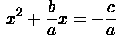Now add the quantity (b/2a)^2 to both sides of the equation: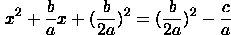The left hand side is a perfect square and right hand side simplified: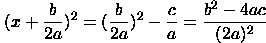Upon taking the square root of both sides and being careful to take both the positive and negative square root, we have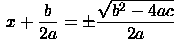Thus, we have derived the quadratic formula: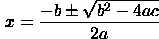Separating these two results, moving quantities to one side and multiplying yields the factorization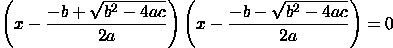Example

When factoring a quadratic, there are four basic methods:

• Factoring by sight (trial and error)
• Following a variant of the completing the square procedure for the given equation

The method you use is irrelevant; how well you use it is relevant. However, for more complicated quadratics the first method is very unappealing. Let's factor x^2+3x+2=0. You are likely familiar with this and can factor it by trial and error; its factorization is (x+1)(x+2)=0. If we did not know this, we could apply the quadratic formula: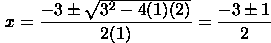This yields x=-2 and x=-1 which means the factorization is (x+1)(x+2) =0. Using a variant of the completing the square method, we add and subtract the familiar quantity (b/2a)^2=9/4 in order to complete the square:By design the first three terms factor into (x+3/2)^2 which means we have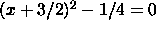This now factors as [(x+3/2)+1/2][(x+3/2)-1/2]=0 which means the factorization is (x+2)(x+1).

Example

Factor: 18x^2-9x-2=0.

This is not a problem conducive to trial and error. So, we will just apply the quadratic formula: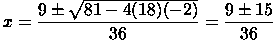Thus, the solutions are x=-1/6 and x=2/3. So this polynomial factors into (x+1/6)(x-2/3)=0, or after multiplying through by 18 to remove the fractions 6(x+1/6)3(x-2/3)=(6x+1)(3x-2)=0.

For higher order polynomials, the goal is to get down to a quadratic that can be easily factored. Consider the polynomial of degree n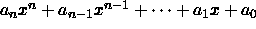Let p be a factor of a_n and q be a factor of a_0. Then px+q may be a factor of the polynomial. To find out, use long division or synthetic division to see if it evenly divides the polynomial. (If you are not familiar with long or synthetic division of polynomials, see the example below, consult an algebra text, or see your professor or T.A.) If it does not divide evenly, try a different factor of a_n and/or a different factor of a_0. If you are lucky, you will soon find a factor that works. You will then be left with a polynomial of degree n-1. The process continues (hopefully) until all that is left is a quadratic which can be easily factored with the quadratic formula.

Example

Factor: 2x^4+9x^3+6x^2-11x-6=0.

For this polynomial, p=1,2 and q=1,-1,2,-2,3,-3,6,-6. With a little insight and luck, the choice of 2x+1 works (we divide by the 2 before synthetic division):
 1/2 | 2 9 6 -11 -6 - | 1 4 1 -6 ---- ---- ---- ---- ---- ---- ---- 2 8 2 -12 0

(The zero in the bottom left hand corner indicates there is no remainder). We now have (x+1/2)(2x^3+8x^2+2x-12)=(2x+1)(x^3+4x^2+x-6). Our new p and q values for the remaining cubic are p=1, q=1,-1,2,-2,3,-3,6,-6. More luck gives x+3 as the next factor:
 3 | 1 4 1 -6 - | 3 3 -6 ---- ---- ---- ---- ---- ---- 1 1 -2 0

We now have (2x+1)(x+3)(x^2+x-2)=(2x+1)(x+3)(x+2)(x-1) =0 and the factorization is complete.

You are likely familiar with the process of finding a common denominator in order to add/subtract fractions with different denominators. For example, by multiplying by cute forms of 1, we can do the following: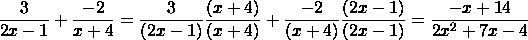You might not be familiar with the reverse process of taking a fraction (with a polynomial of degree greater than 1 in the denominator) and splitting it into a sum of "simple" fractions of the form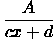The method for doing this is called the method of partial fractions. An example will be the most instructive. Let's use the method of partial fractions to break up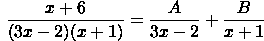Well, we wish to solve for A and B so that the equality holds. To do this find a common denominator on the right and then set the numerators equal: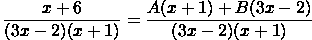which implies x + 6 = Ax + A + 3Bx - 2B. Setting the coefficients of the x terms equal and the constants equal gives A+3B=1 and A-2B=6. Solving this system of two equations and 2 unknowns gives A=4 and B=-1. So our solution is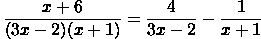If the polynomial in the denominator does not factor completely into linear terms over the real numbers like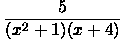then we use the partial fraction decomposition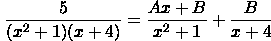Notice the linear polynomial in the numerator of the fraction with the unfactored quadratic in the denominator. (If you are willing to factor over the complex numbers then this second method is not needed - the first method will always work.) The remainder of the process is the same:

• Find a common denominator on the right
• Set the numerators equal
• Set the coefficients of the different powers of x equal
• Solve the system of equations for the unknown constants

Also note that the process of partial fraction decomposition is designed for fractions with a polynomial of lesser degree in the numerator than the denominator.  For problems where the opposite is true, long (or synthetic) division must be carried out first and then the method of partial fractions used on the remainder term (if needed).

Written by Kevin Thompson